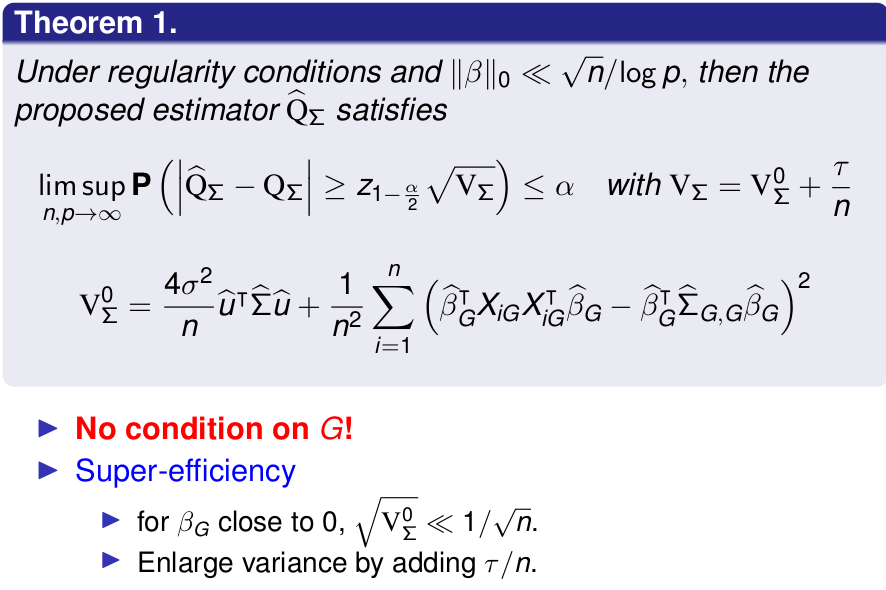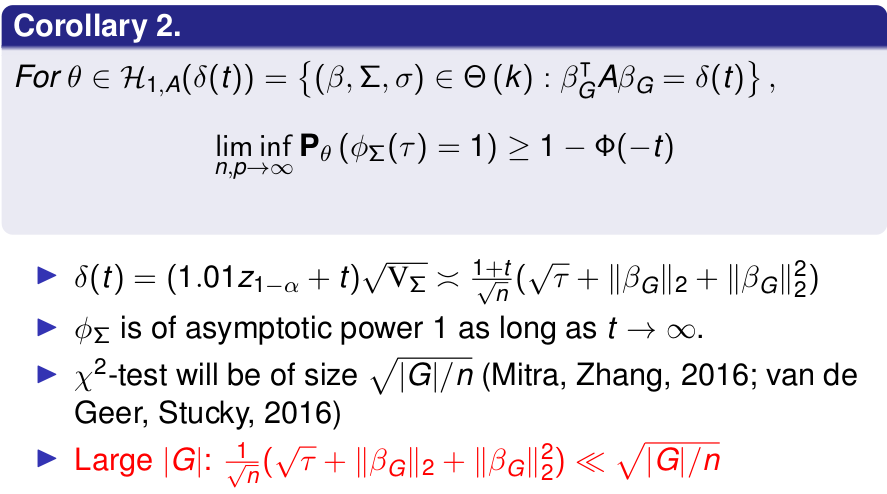# Group Inference in High Dimensions

##### Posted on Dec 17, 2019 (Update: Jan 02, 2020)
Tags: High-Dimensional

This post is based on the slides for the talk given by Zijian Guo at The International Statistical Conference In Memory of Professor Sik-Yum Lee

## Group Inference

High-dimensional linear regression

$y_i = X_{i\cdot}^T\beta + \epsilon_i, \qquad \text{for }1\le i\le n\,,$

where $X_{i\cdot},\beta\in\IR^p$.

• high dimension: $n » p$
• sparse model: $\Vert \beta\Vert_0 « n$

For a given set $G\subset \{1,2,\ldots,p\}$, group significance test is

$H_0: \beta_G = 0\,,$

where $\beta_G = \{\beta_j:j\in G\}$.

## Group Inference vs. Quadratic Functional

The null $H_0:\beta_G=0$ can be written as

$H_{0,A}: \beta_G^TA\beta_G=0\,,$

for some positive definite matrix $A\in\IR^{\vert G\vert \times \vert G\vert}$

Here are two special cases:

• $H_{0,\Sigma}$
• $H_{0, I}$

## Group vs. Individual Significance

For a group of highly correlated variables,

1. It is ambitious to detect significant single variable $\beta_i$ due to inaccurate estimator of $\beta_i$.
2. Significance and high correlation: significant variables can treated as non-significant
3. The group significance

### Hierarchical Testing (Meinshausen, 2008)

Divide variables into sub-groups + group significance

• Variables inside a group tend to be highly correlated
• Between groups, not highly correlated

## Other Motivation I: Interaction Test

Model with interaction:

$y_i = X_{i\cdot}^T\beta + D_i(\gamma_0 + X_{i\cdot}^T\gamma) + \epsilon_{i\cdot}$

To test $H_0:\gamma = 0$ is equivalent to test

$H_0: \eta_G = 0$

where

$y_i = W_{i\cdot}^T\eta + \epsilon_{i\cdot}$

with $W_i = (D_iX_{i\cdot}^T, 1, X_{i\cdot}^T)^T$ and $\eta = (\gamma^T, \gamma_0, \beta^T)^T$.

## Other Motivation II: Local Heritability in Genetics

The proportion of variance explained by a subset of genotypes indexed by the group G, which is the set of SNPs located in on the same chromosome. The local heritability is defined as

$\beta_G^T\Sigma_{G,G}\beta_G = \E\vert X_{i,G}^T\beta_G\vert ^2\,.$

## Goal

Inference for $Q_\Sigma = \beta_G^T\Sigma_{G,G}\beta_G$.

## Bias Correction

Initial estimators

• $\hat \beta = \argmin_{\beta\in\IR^p} \frac{1}{2n}\Vert y-X\beta\Vert_2^2 + \lambda\Vert \beta\Vert_1$
• $\hat\Sigma = \frac 1nX^TX$

Decompose $\hat\beta_G^T\hat\Sigma_{G,G}\hat\beta_G - \beta_G^T\Sigma_{G,G}\beta_G$ as

$-2\hat\beta_G^T\hat\Sigma_{G,G}(\beta_G-\hat\beta_G) + \beta_G^T(\hat \Sigma_{G,G}-\Sigma_{G,G})\beta_G - (\hat\beta_G-\beta_G)^T\hat\Sigma_{G,G}(\hat\beta_G-\beta_G)\,.$

Then estimate $\hat\beta_G^T\hat\Sigma_{G,G}(\beta_G-\hat\beta_G)$ and correct $\hat\beta_G^T\hat\Sigma_{G,G}\hat\beta_G$.

It recalls me the decomposition of $\tilde\alpha$ in the post Optimal estimation of functionals of high-dimensional mean and covariance matrix

## Construction of Projection Direction

For any $u\in \IR^p$,

$u^T\frac 1nX^T(y-X\hat\beta) - \hat\beta_G^T\hat\Sigma_{G,G}(\beta_G-\hat\beta_G) = \frac 1nu^TX^T\epsilon + \left[ \hat\Sigma u - \left(\hat\beta_G^T\hat\Sigma_{G,G} \; 0 \right)^T \right]^T(\beta -\hat\beta)\,.$
• $\Vert \beta - \hat\beta\Vert_1$ is small
$\left\vert \left[ \hat\Sigma u - \left(\hat\beta_G^T\hat\Sigma_{G,G} \; 0 \right)^T \right]^T(\beta -\hat\beta)\right\vert \le \Vert\beta - \hat\beta\Vert_1 \left\Vert \hat\Sigma u -\left(\hat\beta_G^T\hat\Sigma_{G,G} \; 0\right)^T \right\Vert_\infty$
• Minimize/Constrained $u^T\hat\Sigma u$ and
$\Vert \hat\Sigma u -\left( \hat\beta_G^T\hat\Sigma_{G,G}\; 0\right)^T\Vert_\infty = \max_{1\le j\le p}\left \vert\langle e_j, \hat\Sigma u - \left(\hat\beta_G^T\hat\Sigma_{G,G}\; 0\right)^T\rangle\right\vert\,.$

Initial proposal

$\hat u = \argmin u^T\hat\Sigma u$

s.t.

$\max_{w\in \cC_0}\left\vert \langle w, \hat\Sigma u - \left(\hat\beta_G^T\hat\Sigma_{G,G}\; 0\right)^T\rangle\right\vert \le \Vert \hat\Sigma_{G,G}\hat\beta_G\Vert_2\lambda_n$

and

$\cC_0 = \left\{e_1,\ldots,e_p\right\}\,.$

Replace $\cC_0$ with

$\cC = \left\{ e_1,\ldots, e_p, \frac{1}{\Vert \hat\Sigma_{G,G}\hat\beta_G\Vert_2}\left( \hat\beta_G^T\hat\Sigma_{G,G}\; 0 \right)^T \right\}\,,$

then it works for any $\vert G\vert$.

The Constrain Variance becomes

$\hat Q_\Sigma = \hat\beta_G^T\hat\Sigma_{G,G}\hat\beta_G + \frac 2n\hat u^TX^T(y-X\hat\beta)\,.$

## Inference Procedure

Estimate the variance of the proposed estimator $\hat Q_\Sigma$ by $\hat V_\Sigma(\tau)$. The decision rule (test) is

$\phi_\Sigma(\tau) = 1\left(\hat Q_\Sigma \ge z_{1-\alpha}\sqrt{\hat V_\Sigma(\tau)}\right)$

and the confidence interval is

$\text{CI}_\Sigma(\tau) = \left(\hat Q_\Sigma - z_{1-\alpha/2}\sqrt{\hat V_\Sigma(\tau)}, \hat Q_\Sigma + z_{1-\alpha/2}\sqrt{\hat V_\Sigma(\tau)}\right)\,.$

## Theoretical Justification## Size and Power